# Kratky analysis¶

A Kratky plot is a plot of $$q^2I(q)$$ vs. q. Kratky plots can qualitatively assess the flexibility and/or degree of unfolding in samples. Unfolded (highly flexible) proteins should have a plateau in the Kratky plot at high q, while compact, globular proteins will have a bell-shaped (Gaussian) peak. A partially unfolded (flexible) protein may have a combination of the bell-shape and plateau, or a plateau that slowly decays to zero.

Normalized Kratky plots are plots of $$q^2I(q)/I(0)$$ vs. q. This normalizes scattering profiles by mass and concentration. Dimensionless Kratky plots are presented as either $$(qR_g)^2I(q)/I(0)$$ vs. $$qR_g$$or $$(q^2V_c)I(q)/I(0)$$ vs. $$q(V_c)^{1/2}$$. These dimensionless plots can provide semi-quantitative analysis of flexibility and disorder. More information about can be found here and references therein: https://www.bioisis.net/tutorials/21.

A video version of this tutorial is available:

The written version of the tutorial follows.

1. If you haven’t already, save the loaded scattering profiles, remove all loaded profiles from RAW, and reload your saved GI and Lysozyme profiles, as described in the previous section.

2. Put the top plot on Kratky axes.

• Tip: Right click on the plot to change the plot type.
3. Show only the top plot by clicking on the 1 in the plot control bar below the plots.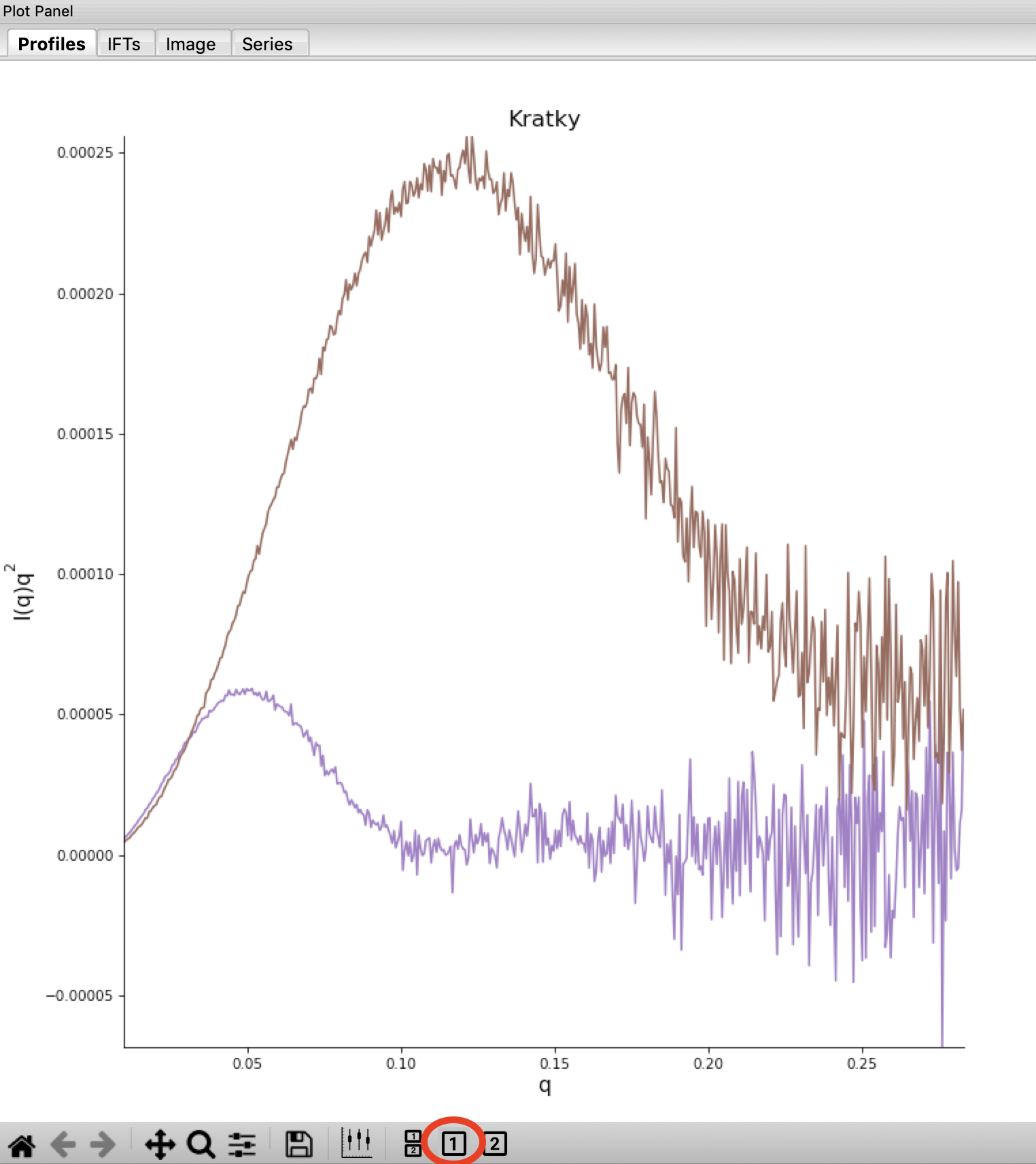4. Both GI and lysozyme show the classic bell shape, indicating they are completely folded.

• Warning: Bad buffer subtraction can also result in a Kratky plot that appears to show some degree of flexibility. Excellent buffer subtraction is required for accurately analysis with this technique.
5. Load the two scattering profiles in the Tutorial_Data/flexibility_data folder.

• Note: The unfolded.dat file is the scattering profile of an unfolded lysine riboswitch. The partially_folded.dat file is same lysine riboswitch, but in the biologically functional configuration. The data were downloaded from the BIOISIS database, and has the BIOISIS ids of 2LYSRR and 3LYSRR.
6. SAXS data can be presented on an arbitrary scale, which is why these two profiles have intensity that is much larger than the lysozyme and GI data (which is on an absolute scale). Use the triangle button for each item in the Profiles list to show more options. Hide one of the newly loaded data sets, and adjust the scale factor on the other until you can comfortably see it and your lysozyme and GI data. Repeat the scale adjustment for the other data set.

• Tip: The up and down arrows will only adjust the last digit of the scale factor.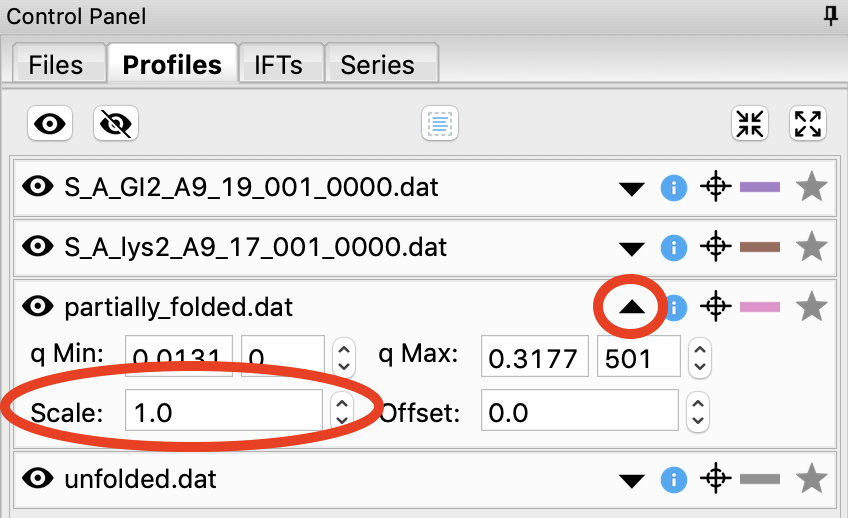7. Kratky analysis can also be done on normalized or dimensionless data. RAW supports normalization by I(0), and non-dimensionalization by Rg and Vc (the volume of correlation).

8. Select all four loaded scattering profiles, right click, and select the Dimensionless Kratky Plot option.

9. Normalized and dimensionless Kratky plots require Guinier analysis to be done. If one or more profiles are missing this information, RAW will show the following window. You can either cancel, and do the fits manually, or you can proceed with RAW’s automatic determination.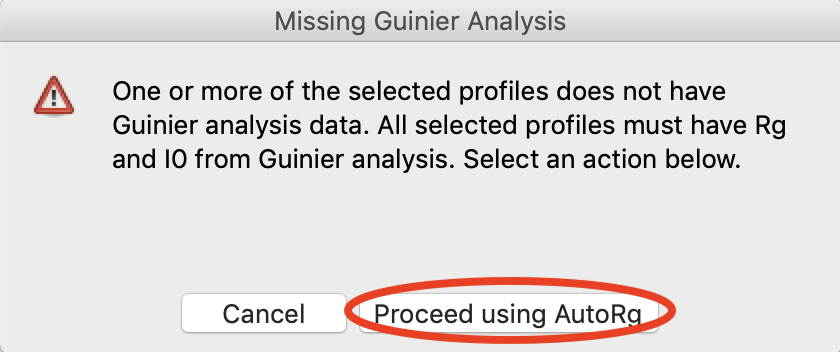10. Click the Proceed using AutoRg button to proceed to the Dimensionless Kratky Plot window using RAW’s automatic fitting for Rg.

11. By default, the plot is the Dimensionless Rg plot. Use the drop-down “Plot” menu at the top to select the Normalized (by I(0)) and Dimensionless Vc plots.

• Tip: The dashed gray lines on the Dimensionless (Rg) plot are guidelines for a globular protein. For a globular protein the peak position should be at $$qR_g=\sqrt{3}\approx 1.73$$, while peak height should be $$3/e\approx 1.1$$.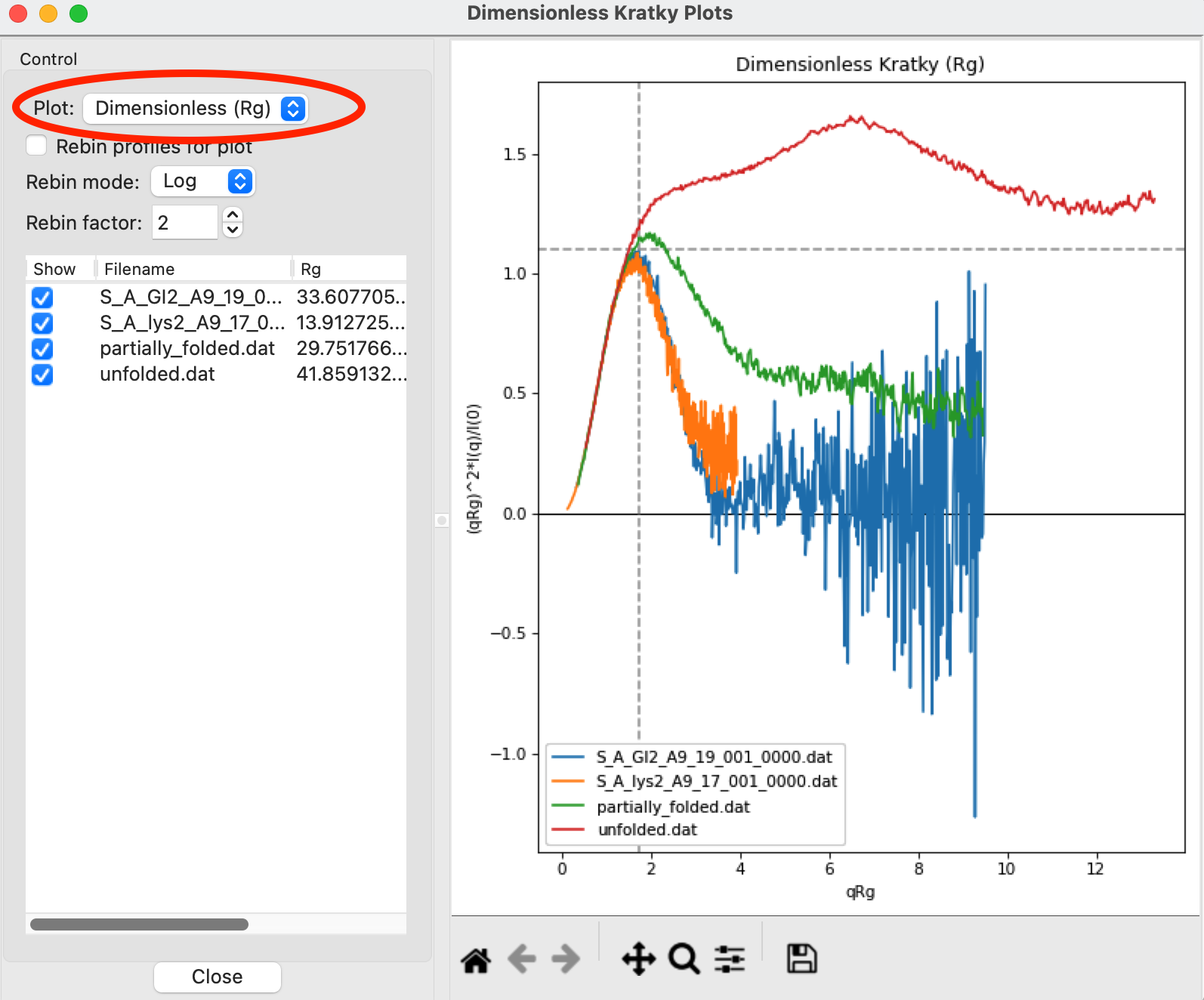12. Return to the Dimensionless Rg plot. Use the check boxes to hide the partially_folded and unfolded data sets on the plot. Note that both the lysozyme and GI data look very similar on this plot, showing they have similar shapes and (lack of) flexibility.

• Tip: You can click on the colored line in the Color column to change the color of an item on the plot.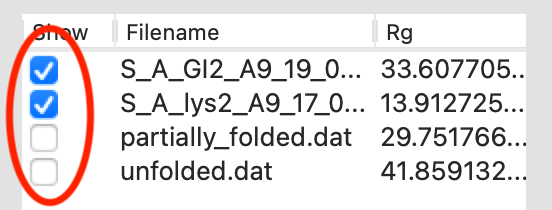13. Right click on the plot and select “Export Data As CSV” to save the dimensionless data for further processing or plotting with another program.

14. Click the Close button to close the Dimensionless Kratky Plot window.